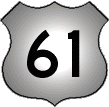# 61

This number is a prime.

Just showing those entries submitted by 'Rivera': (Click here to show all)The sum of the first n primes type 4k+1 is equal to 2^x, just for two n values, i.e., n=4 and n=8, producing the following sums: 5+13+17+29=64=2^6 and 5+13+17+29+37+41+53+61=256=2^8. Will this ever be satisfied again for another pair of n and x values? [Rivera]Prime numbers that end with "61" occur more often than any other 2-digit ending among all primes less than one billion. [Rivera]